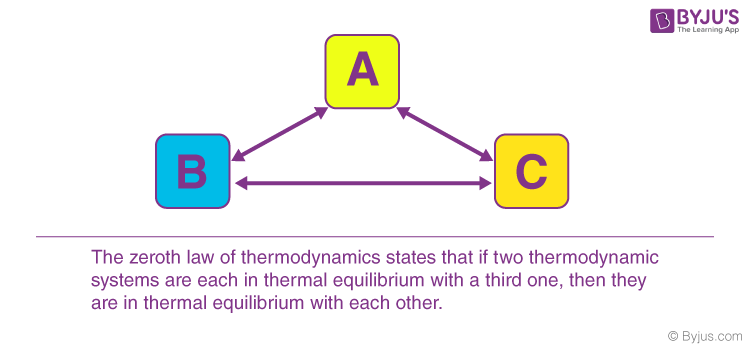Win up to 100% scholarship on Aakash BYJU'S JEE/NEET courses with ABNAT Win up to 100% scholarship on Aakash BYJU'S JEE/NEET courses with ABNAT

# Zeroth Law of Thermodynamics

Zeroth Law of Thermodynamics is one of the four laws of thermodynamics. The credit for formulating the law goes to Ralph H. Fowler. Interestingly, the zeroth law of thermodynamics was actually developed much later than the original three laws. However, there was some confusion regarding the nomenclature, whether it should be named the fourth law or some other name. The complication arose because the new law gave a much clearer definition of the temperature and basically replaced what the other three laws had to state. Finally, Fowler came up with a name to end this conflict.

The zeroth law of thermodynamics frames an idea of temperature as an indicator of thermal equilibrium.

## What Is Zeroth Law of Thermodynamics?

When a body, ‘A’, is in thermal equilibrium with another body, ‘b’, and also separately in thermal equilibrium with a body ‘, C’, then body, ‘B’ and ‘C’, will also be in thermal equilibrium with each other. This statement defines the zeroth law of thermodynamics. The law is based on temperature measurement.There are also various ways to state the zeroth law of thermodynamics. However, in simple terms, it can be said, “Systems that are in thermal equilibrium exist at the same temperature”.

Zeroth law of thermodynamics takes into account that temperature is something worth measuring because it predicts whether heat will transfer between objects or not. This is true regardless of how the objects interact. Even if two objects are not in physical contact, heat can still flow between them by means of the radiation mode of heat transfer. On the other hand, the zeroth law of thermodynamics states that if the systems are in thermal equilibrium, no heat flow will take place.

## Thermal Equilibrium

Temperature is a property that distinguishes thermodynamics from other sciences. This property can distinguish between hot and cold. When two or more bodies at different temperatures are brought into contact, then after some time, they attain a common temperature, and they are said to exist in thermal equilibrium.

Systems are said to be in thermal equilibrium if there is no heat transfer, even if they are in a position to transfer heat, based on other factors. For example, if we put food in the refrigerator overnight, then the food is in thermal equilibrium with the air of the refrigerator. Heat no longer flows from food to the air or from the air to the food, and this state is known as thermal equilibrium.

## Zeroth Law of Thermodynamics Example and Applications

The zeroth law of thermodynamics is important for the mathematical formulation of thermodynamics or, more precisely, to state the mathematical definition of temperature. This law is mostly used to compare the temperatures of different objects.

If we want to measure the accurate temperature, a reference body is required, and a certain characteristic of the body changes with temperature. The change in the characteristic may be taken as an indication of a change in temperature. The selected characteristic is known as thermodynamic property.

Nonetheless, the most common application of the zeroth law of thermodynamics can be seen in thermometers. We can observe the zeroth law in action by taking a very common thermometer having mercury in a tube. As the temperature increases, this mercury expands since the area of the tube is constant. Due to this expansion, the height is increased. Now, the increase in the height of the mercury label shows the changes in temperature and basically helps us to measure it.

There are different kinds of thermometers that can be used depending on their thermometric property, and they are as follows.

 Thermometer Thermometric Property Constant volume gas thermometer Pressure Constant pressure gas thermometer Volume Electrical resistance thermometer Resistant Thermocouple Thermal e.m.f. Mercury-in-glass thermometer Length

Similarly, another example of the zeroth law of thermodynamics is when you have two glasses of water. One glass contains hot water, and the other contains cold water. Now, if we leave them on the table for a few hours, they will attain thermal equilibrium with the temperature of the room.

#### Thermodynamics Important Topics#### Thermodynamics Important Questions#### Thermodynamics Top 7 Questions#### Thermodynamics JEE Solutions#### Thermodynamics JEE Main Important Questions## Frequently Asked Questions on Zeroth Law of Thermodynamics

Q1

### Define thermal equilibrium.

Two bodies or systems in contact are said to be in thermal equilibrium if both are at the same temperature.

Q2

### Define Zeroth Law of Thermodynamics.

If two systems, A and B, are separately in thermal equilibrium with a third system, C, then A and B are also in thermal equilibrium with each other.

Q3

### Define the temperature of a system.

The temperature of a system is a physical quantity that determines whether the system is in thermal equilibrium with another system in its contact or not.

Q4

### What is internal energy?

The internal energy of a system is defined as the sum of the kinetic energies of the constituent particles of the system.

Test your Knowledge on Zeroth law of thermodynamics